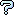All about flooble | fun stuff | Get a free chatterbox | Free JavaScript | Avatarsperplexus dot infoArithmetic and Geometric Pandigital (Posted on 2009-07-16)Each of P, Q, R, S and (P+S), with P < Q < R < S, is a non leading zero 10-digit base ten positive integer containing each of the digits from 0 to 9 exactly once. It is known that R is the arithmetic mean of P and S, and Q is the geometric mean of P and S.

Determine the minimum value of P and the maximum value of S.

 No Solution Yet Submitted by K Sengupta No RatingComments: ( Back to comment list | You must be logged in to post comments.)re: Considerations which might reduce programming| Comment 7 of 11 |(In reply to Considerations which might reduce programming by brianjn)

Why must P and S be perfect squares? Is it merely because when multiplied together they must produce a perfect square (the square of Q)? But two numbers that are not perfect squares can multiply out to a perfect square. Or is there a reason that I'm missing, that requires that P and S be perfect squares?
 Posted by Charlie on 2009-07-23 16:48:44Please log in:

 Search: Search body:
Forums (1)